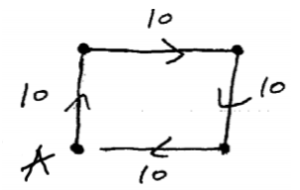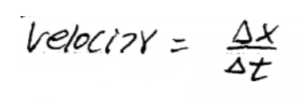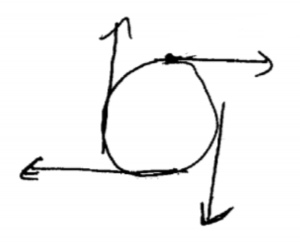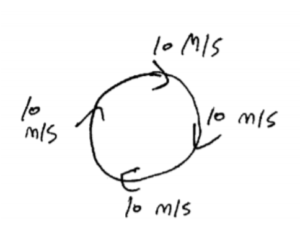Blog / Speed Velocity Average And Instantaneous

### Speed, velocity (average and instantaneous)

Written by Chau Pham on Feb 26, 2020

Velocity the rate of change of displacement with respect to change in time, velocity is a vector with magnitude and direction. Speed is the magnitude of velocity.

Take this example into consideration:If you start travelling from point A: The distance travelled would be 40 (units of distance), while the displacement is 0. The displacement is a vector which depends on the start and end point. In this example, the start and end point is the same, hence displacement is 0. To have a velocity, you must have a change in díplacement.

Average velocity is displacement over a period of time:As an object moves, its direction could change. The direction of the instantaneous velocity of a particle is always tangent to the particle’s path at the particle’s position.In uniform circular motion, you can have the same speed with different velocities at every point (due to the changing directions).### KEY TAKEAWAYS

Key Points:

• Velocity is a vector which represents the change in displacement with respect to change in time. Speed is the magnitude of velocity.
• In uniform circular motion, magnitude of velocity is constant while its direction changes.

Key Terms:

• Displacement: change of position
• Average velocity: displacement over a period of time.
• Instantaneous velocity: direction is always tangent to the particle’s path at the particle’s position.
• Uniform circular motion: movement of an object along the circumference of a circle with constant speed and changing direction.

Billing Information